# Frank Solutions for Chapter 6 Electrolysis Class 10 Chemistry ICSE

1. Define the following terms :
(a) Electrolysis
(b) Electrolyte
(c) Non-electrolyte
(d) Cation and anion

(a) Electrolysis: It is the process of decomposition of the electrolyte in the molten or aqueous state by discharge of ions at the electrodes on passage of an electric current.
(b) Electrolyte: It is a compound which either in aqueous solution or in the molten state allows an electric current to pass through it and is accompanied by discharge of ions and finally into neutral atoms at the two electrodes. For example : Hydrochloric acid.
(c) Non-electrolyte: They are substances which do not conduct electricity in the fused or aqueous state. They contain only molecules and do no ionize. For example : Petrol.
(d) Cation and anion :
Cation: Positively charged ions are called cations. For example : Na+
Anion: Negatively charged ions are called anion. For example : Cl-

2. From the given list : NaCl, NaOH, H2O (pure), NH4OH, urea, dil. H2SO4 , glucose, acetic acid, H2CO3 , select :
(a) Substances which will behave as strong electrolytes.
(b) Substances which will behave as weak electrolytes.
(c) Substances which are non - electrolytes.

(a) Substances which will behave as strong electrolytes :
NaCl, NaOH, H2O(pure), dil. H2SO4
(b) Substances which will behave as weak electrolytes : NH4OH, acetic acid H2CO3
(c) Substances which are non - electrolytes : urea, glucose

3. Write the differences between with examples :
(a) A strong electrolyte and a weak electrolyte
(b) Electrolytic dissociation and ionization

(a) Difference between a strong electrolyte and a weak electrolyte

 S. No. Characteristics Strong electrolyte Weak electrolyte 1. Dissociation into ions in aqueous solution Almost complete Partial and not much 2. Species present in solution Almost only free ions Both ions and undissociated molecules 3. Number of ions present in solution Very large Only limited in numbers 4. Quantity of current conducted Large amounts, a bulb glows brightly Only small amounts, a bulb glows dimly 5. Examples HCl, NaOH, NaCl CH3COOH, NH4OH

(b) Electrolytic dissociation and ionization

 S. No. Ionization Electrolytic dissociation 1. Formation of positively or negatively charged ions from molecules which are not initially in the ionic state. Separation of ions which are already present in an ionic compound. 2. Polar covalent compounds show ionization Electrovalent compounds show dissociation 3. For example : HCl, For example : KCl,

4. Explain electrolysis of lead bromide.

A steel rod is connected to the negative terminal and a graphite rod to positive terminal of a battery. A silica crucible is filled to about two thirds with solid lead bromide and the rods are dipped into it and is then melted by heating it over a Bunsen burner.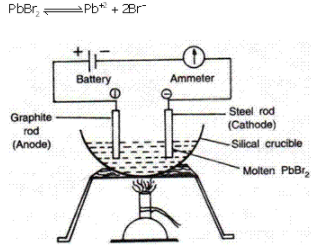Reaction at cathode : Lead ions are positively charged they get discharged at cathode. It receives two electrons from cathode and changes to atom of lead and get deposited at the cathode.
Pb+2 + 2e- Pb
Reaction at the anode : Bromide ions, being negatively charged discharge at anode. It loses its only electron and becomes an atom of bromine. Thus the atoms of bromine combine in pairs to form molecules of bromine which escape as red vapours, at the anode.5. Give three application of electrolysis.

Three applications of electrolysis are :
(i) Electroplating with metals
(ii) Electro refining of  metals
(iii) Extraction of metals

6. State your observations for the following electrolytic reactions :
(a) Solid copper sulphate is electrolysed between platinum electrodes.
(b) Aqueous copper sulphate is electrolysed between platinum electrodes.
(c) Aqueous copper sulphate is electrolysed between copper electrodes.

(a) When solid copper sulphate is electrolysed between platinum electrodes the electrolytic reaction will not take place as no electrolytes are formed in solid state.
(b) When the electrolysis of copper sulphate (aqueous) is electrolysed between platinum electrodes the following reaction follows :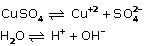Reaction at cathode : Copper ions and hydrogen ions migrate towards the cathode.
Cu+2  ions being lower in the electrochemical series are preferentially discharged to hydrogen ions to form neutral copper atoms at platinum cathode.Reaction at anode : Sulphate ions and hydroxyl ions migrate towards the anode. Hydroxyl ions are preferentially discharged to sulphate ions at anode, to form neutral particles of OH- . The electrically neutral hydroxyl reacts among themselves to give water and oxygen.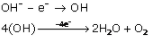(c) When Aqueous copper sulphate is electrolysed between copper electrodes then the ions formed areReaction at the cathode : Copper and hydrogen ions both being positively charged migrate towards the cathode. Copper ions are discharged in preference to hydrogen ions. Copper gains two electrons from the cathode and changes into an atom of copper. The atoms of copper are deposited at the cathode. The atoms of copper are deposited at the cathode ad form a layer of pink copper metal which gradually turns reddish brown.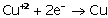Reaction at the anode : Anode receives electrons from the ions and supplies them to the cathode. The atoms of copper from the anode changes into ions of copper which go into the solution and the electrons liberated in this change are taken up by the anode.Thus for every copper ion discharged at the cathode, an ion of copper is formed at the anode which goes into the solution. Thus the atoms of copper are deposited at the cathode. the cathode becomes thicker and the atoms of copper from the anode change into ions of copper, the anode becomes thinner.Therefore there is transference of copper atoms from anode to cathode.

7. Explain, why electrolysis is an example of redox reaction ?

Redox reaction is one in which oxidation and reduction occurs simultaneously.
Similarly in case of electrolysis:
At cathode: The cations gain electron and become neutral. As the electrons are gained the ion is said to be reduced.
At anode: The anions lose electron to form neutral atoms. As the electrons are lost the ion is said to be oxidized.
Hence in electrolysis also the oxidation and reduction occurs hence it is an example of redox reaction.

8. State the factors that influence the preferential discharge of ions at the electrodes.

The following are the factors that Influence the preferential discharge of Ions at the electrode:

1. Position of the metallic ion in electrochemical series: If all the factors remain the same, an ion placed lower In the electrochemical series gets preferentially discharged at the respective electrode In comparison to all those ions, which are placed above it in the series.
2. Concentration of ions in the electrolyte: Higher the concentration of negative ion in the electrolytic solution, greater is Its probability of being discharged at the anode.
3. iii) Nature of the electrode: If the electrode used is inert i.e. made of less reactive material such as graphite, platinum etc., the electrode does not play any role in deciding the preferential discharge of an ion at it.
If the electrode used is active i.e. made of active material such as Cu, Ag, Ni etc. it takes part in the electrode reaction and plays an important role in deciding the ions which will preferentially be discharged. In such a case, anions migrate to the anode but do not get discharged Instead the active anode Itself loses electrons and form Ions.

9. With reference to the electrolysis of acidulated water, answer the following :
(a) Why distilled water is a non - electrolyte ?
(b) What is the electrolytic cell called ?
(c) State what you would observe at the (i) Cathode (ii) Anode
(d) Summarize the electrode reactions.
(e) Why is electrolysis of acidulated water considered as an example of catalysis ?

(a) Distilled water is a non electrolyte because it does not contain any ions.
(b) The electrolytic cell used in electrolysis of acidulated water is called Hoffmann's Apparatus.

(c) (i) At cathode: Hydrogen ions are H+ ions. They migrate to the cathode and discharge there. The ions gain electrons from the cathode to form atoms of Hydrogen which combine in pairs to form molecules of Hydrogen.(ii) At anode: Sulphate ions and hydroxyl ions are the anions present in the solution. Both migrate to the anode. OH' ions are discharged in preference to sulphate ions. The OH- ions loose their electrons and become electrically neutral particles of OH, which react among themselves to give water and oxygen.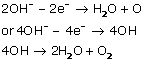(d) Electrolytic Cell: Hoffmann Voltameter
Electrolyte: Acidified water
Electrode: Cathode - Platinum Foil,  Anode - Platinum Foil
Dissociation of acidified water: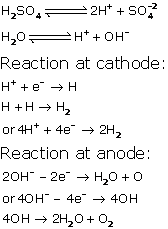(e) Electrolysis of acidulated water is considered as an example of catalysis because the reaction is catalyzed by acids.

10. Make a neatly labeled sketch to show how a brass spoon can be plated with silver. Explain your choice of electrolyte used.

In order to get a spoon plated with silver a solution of sodium silver silver cyanide can be taken as electrolyte. If silver nitrate was chosen as electrolyte then the deposition of silver will be very fast and hence not very uniform and smooth,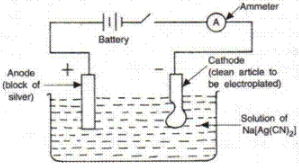11. Explain the terms :
(i) Electro refining
(ii) Electro metallurgy
(iii) Anode mud

(i) Electro refining : It is the process of refining the impure metals through the use of electric current through the use of electric current of electrolysis.
(ii) Electro metallurgy : It is the process of extraction of metal from its ore through the use of electric current.
(iii) Anode mud : During electro refining some impurities which are insoluble fall down near the anode and is known as anode mud.

12. Fill in the blanks :
(a) The metal plate trough which current enters into an electrolyte is called ____. It has _____ of electrons.
(b) The metal plate through which ____ leaves from an electrolyte is called ____. It has ____ of electrons.
(c) The ions which discharge on the negative electrode during electrolysis ____ electrons. Thus, the ions are said to be _____ .
(d) The ions which discharge on the positive electrode during electrolysis ____ electrons. Thus, the ions are said to be ____.
(e) Hydrogen and metallic ions are ____ because they ____ electrons
(f) Non - metallic ions are _____ because they ____ electrons.

(a) Anode, lack
(b) gain, Cations
(c) Loose, anions
(e) Electropositive, loose
(f) Electronegative, gain

13. (a) Al+3 , Cu+2 , Na+ , Zn+2  ions are present in aqueous solution, such that the concentration of ions is same. Write the order of discharge of ions.
(b) Amongst the OH- ions and Br-  ions which are likely to discharge first ?

(a) The order of discharge of ions is Cu+2, Zn+2, Al+3 because as the concentration is same, an ion placed lower in electrochemical series get preferentially discharged.
(b) OH- is likely to discharge first in comparison to Br-  ions.

14. How electrolysis can be used in extraction of aluminum ? Why aluminum cannot be reduced by conventional reducing agents ?

Aluminium is extracted by the electrolysis of fused pure alumina dissolved in fused mixture of cryolite and fluorspar . This mixtures lowers the melting point of alumina and increases the electrical conductivity and the electrolysis Is carried out in an Iron tank lined with gas carbon. This lining of a gas carbon serves as cathode. The anode consists of a number of carbon rods which dip in fused electrolyte. On passing electric current following reaction takes place.Certain metal oxides are highly stable and so cannot be reduced by conventional reducing agents like coke, carbon monoxide or hydrogen. They are extracted from their oxides or salts by the electrolysis in fused state. For e.g., metals like aluminium, sodium, potassium, magnesium, titanium, calcium etc. are extracted by this method.

15. Explain, why during the electrolysis of copper sulphate using copper electrodes, the colour of solution does not fade?

During the electrolysis of copper sulphate using copper electrode, the number of copper ions remains same as that for evey copper ions discharged at the cathode, an ion of copper is formed at the anode which goes in the solution. Thus the colour intensity does not change.

16. How is impure copper purified by electrolysis ? Explain.

Electrolytic refining is used for the purification of copper . In this case
Cathode: Pure copper strip
Anode: Impure copper
Electrolyte: A solution of copper sulphate and dilute sulphuric acid
When the current is passed through the electrolyte , the copper ions of the copper sulphate solution are attracted to the cathode where they gain electrons and are deposited on pure copper strips. The impure copper loses electrons and passes into solution as soluble copper ions.17. List out the main applications of electrolysis.

The main application of electrolysis are :
(i) Electroplating with metals
(ii) Electrorefining of metals
(iii) Extraction of metals

18. How is eletrolytic dissociation different from thermal dissociation ?

Electrolytic dissociation is the dissociation of an electrovalent compound into ions in the fused state or in aqueous solution state.
Reversible breakdown of a chemical compound into simpler substances by heating it. The splitting of ammonium chloride into ammonia and hydrogen chloride is an example. On cooling, they recombine to form the salt.

19. A solution of caustic soda (NaOH) in water or when fused, conducts an electric current. What is the similarity in these two cases ?

A Solution of NaOH dissociates by electrolytic dissociation.
And fused NaOH dissociates by thermal dissociation.
The similarity between both of these is that they both liberate same ions.20. Name :
(a) A salt which is a weak electrolyte
(b) A base which is not alkali
(c) An inert electrode and an active electrode
(d) A positively charged not metallic ion
(e) A non-metallic element which is a conductor of electricity.

21. Choose the correct answer from the options given below:

(i) Which one is weak electrolyte?
(a) HNO3
(b) KOH
(c) CuSO4
(d) Cu(OH)2
(c) CuSO4

(ii) Which among the following cations will discharge with ease at cathode?
(a) Na+
(b) Au3+
(c) Cu2+
(d) H+
(b) Au3+

(iii) Which among the following anions will discharge with ease at anode?
(a) Cl-
(b) I-
(c) OH-
(d)(c) OH

(iv) In electrolysis of molten lead bromine anode is made up of
(a) Steel rod
(b) Platinum foil
(c) Glass rod
(d) Graphite rod
(d) Graphite rod

(v) Electrolysis of acidulated water is used in the production of
(a) Hydrogen
(b) Oxygen
(c) Nitrogen
(d) Hydrogen and oxygen
(d) Hydrogen and oxygen

22. The following questions relate to the electroplating of an article with silver.

(a) Name the electrode formed by the article which is to be plated.
(b) What ions must be present in the electrolyte?
(c) What should be the nature of the anode?

(a) The article to be plated must be made Cathode.
(b) The ions of the metal which is to be electroplated must be present in the electrolyte.
(c) The metal to be plated on the article must be made anode. It needs to be periodically replaced.

23. How is the passage of electricity through an electrolyte different from the passage of electricity through a copper wire?

The passage of electricity through an electrolyte occurs through ions furnished by the electrolyte where as the passage of electricity through a copper wire occurs through electrons.

24. The following questions are about electroplating of copper wire with silver.
(a) What ions must be present in the electrolyte?
(b) Of what substance must the anode be made up of?
(c) What will the cathode be made up of?
(d) Write the equation for the reaction which takes place at the cathode.

(a) The ions of the silver must be present in the electrolyte.
(b) Anode should be made of pure clean silver.
(c) The cathode is made of copper wire.
(d) The equation for the reaction which takes place at the cathode is25. Define or explain the term: Electrolysis.

It is the process of decomposition of an electrolyte in the molten or aqueous state by discharge of ions at the electrodes on the passage of an electric current.

26. Why is it necessary to add acid to water before proceeding with electrolysis of 'water'?

Pure water does not conduct electricity because the degree of ionization is low. Thus to make it a good conductor of electricity acid is added to it which will increase the degree of ionization.

27. Give one example of a substance which contains: (i) Ions only (ii) molecules only (iii) both ions and molecules.

Substance which contain
(i) Ions only: HCl
(ii) Molecules only: Petrol
(iii) Both ions and molecules: CH3COOH

28. (a) What is meant by the term 'electrolyte'?
(b) What are the particles present in a compound which is a non- electrolyte?
(c) If an electrolyte is described as a 'strong electrolyte' what does this mean?

(a) Electrolyte is a compound which either in aqueous solution or in molten state allows an electric current to pass through it and is accompanied by discharge of ions and finally into neutral atoms at the two electrodes.
(b) Non- electrolyte are substances which do not conduct electricity in fused or aqueous state. They contain only molecules and do not ionize. For example: petrol, alcohol.
(c) If the electrolyte is described as 'strong electrolyte' it means it completely dissociates into its constituting ions in aqueous solution.

29. The following Question refer to the electrolysis of copper sulphate solution with copper electrodes:
(a) Compare the change in mass of the anode
(b) What is seen to happen to the colour of the copper sulphate solution if platinum electrodes are used? Explain the observation.
(c) What is the practical application of the electrolysis of copper sulphate solution? Briefly, describe one such application.

(a) As for every copper ion discharged at the cathode, an ion of copper is formed at the anode which goes into the solution .Since atoms of copper are deposited at the cathode, the cathode becomes thicker and as the atoms of copper from the anode change into ions of copper, the anode becomes thinner.
(b) When platinum rods are used as electrodes, then x the blue colour of copper sulphate solution fades and sulphuric acid is formed. This is because oxygen is liberated at anode and copper metal is deposited at cathode
(c) Practical application of electrolysis of copper sulphate solution: This is the basis for purification of copper.
Other metals like Zinc, Nickel, Silver .Lead can also be purified.

30. What should be the physical state of lead bromide if it is to conduct electricity?

Lead Bromide should be in the molten state if it has to conduct electricity.

31. What particles are present in pure lead bromide?

Lead Bromide contains only positively charged lead ions and negatively charged bromide ions.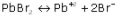32. Write the equations for the reactions, which takes place at the electrodes during the electrolysis of lead bromide?

Equations for the reactions, which takes place at the electrodes : -
At Cathode :33. Choosing only words from the following list, write down the appropriate words to fill in the blanks (a) to (e) below: anions, anode, cathode, cations, electrode, electrolyte, nickel, voltameter.
To electroplate an article with nickel requires an (a) ________ which must be a solution containing (b) ________ions. The article to be plated is placed as the (c) _________of the cell in which the plating is carried out. The (d)________ of the cell is made from pure nickel. The ions that are attracted to the negative electrode and discharged are called (e)________.

(a) Electrolyte
(b) Nickel
(c) cathode
(d) anode
(e) Cations

34. Correct the sentence by adding word(s)

The electrolysis of lead bromide liberates lead at cathode and bromine at anode.

35. If a fused metallic chloride is electrolyzed, at which electrode would the metal be obtained?

When a fused metallic chloride is electrolyzed, the metal is obtained at cathode.

36. Classify the following substances under three headings:
(a) Strong electrolytes
(b) weak electrolytes
(c) non- electrolytes

Acetic acid, ammonium chloride, ammonium hydroxide, carbon tetrachloride, dilute hydrochloric acid, sodium acetate, dilute sulphuric acid.

(a) Strong electrolytes - dilute hydrochloric acid, dilute sulphuric acid, Ammonium chloride
(b) Weak electrolyte - Acetic acid, Ammonium hydroxide
(c) Non-electrolytes - Carbon tetrachloride

37. (a) Write down the words or phrases from the brackets that will correctly fill in the blanks in the following sentences:
(i) Pure water consists entirely of ______ ( ions/ molecules).
(ii) We can expect that pure water _______ ( will / will not) normally conduct electricity.

(i) molecules.
(ii) will not

38. (a) To carry out the so called "electrolysis of water", sulphuric acid is added to water. How does the addition of sulphuric acid produce a conducting solution?
(b) Copy and complete the following sentence :
With platinum electrodes, hydrogen is liberated at the ______and oxygen at the _________ during the electrolysis of acidified water.

(a) When sulphuric acid is added to water it becomes good conductor as addition of sulphuric acid causes dissociation of water molecules into H+ and OH- ions which are then responsible for conduction of electricity by pure water. The water thus obtained is called acidified water.
(b) Cathode, Anode

39. Copy and complete the following table which refers to two practical applications of electrolysis.

 Anode Electrolyte Cathode Silver plating of a spoon Purification of copper Solution of potassium argentocyanide

 Anode Electrolyte Cathode Silver plating of a spoon Purification of copper Plate of pure clean silver Impure Copper Solution of potassium argentocyanide A solution of copper sulphate and dilute sulphuric acid Cleaned article to be electroplated Thin strip of pure copper

40. Complete the sentence by choosing correct words given in brackets.
Electrolysis is the passage of __________ (electricity/electrons) through a liquid or solution accompanied by a __________ ( physical/chemical ) change.

electricity, chemical

41. Element X is a metal with valency 2. Element Y is a non-metal with valency 3.

(a) Write equations to show how X and Y form ions.
(b) If Y is diatomic gas, write the equation for the direct combination of X and Y to form a compound.
(c) Write two applications of electrolysis in which anode diminish in mass.
(d) If the compound formed between X and Y is melted and an electric current passed through the molten compound, the element X will be obtained at the _____ and the Y at the ________of the electrolytic cell. (Provide the missing words).(c) (i) Electroplating of metals.
(ii) Electrorefining of metals.
(d) If the compound formed between X and Y is melted and an electric current passed through the molten compound, the element X will be obtained at the cathode and y at the anode of the electrolytic cell.

42. (a) What kind of particles will be found in a liquid compound which is a non- electrolyte?
(b) If HX is a weak acid, what particles will be present in its dilute solution apart from those of water?
(c) Cations are formed by _______ (loss/ gain) of electrons and anions are formed by ________ (loss/gain) of electrons.
(Choose the correct words to fill in the blanks.)
(d) What ions must be present in a solution used for electroplating a particular metal?
(e) Explain how electrolysis is an example of Redox reaction.

(a) Molecules are found in a liquid compound which is a non-electrolyte.
(b) Non ionized molecules; H+ and X- particles will be present in dilute solution.
(c) Loss, Gain
(d) The ions of the metal which is to be electroplated on the article must be present in a solution.
(e) Redox reaction is one in which oxidation and reduction occurs simultaneously.
Similarly in case of electrolysis:
At cathode: The cations gain electron and become neutral. As the electrons are gained the ion is said to be reduced.
At anode: The anions lose electron to form neutral atoms. As the electrons are lost the ion is said to be oxidized.
Hence, in electrolysis also the oxidation and reduction occurs hence it is an example of Redox reaction.

43. (i) Explain, why copper though a good conductor of electricity is, a non- electrolyte.
(ii) Name the gas released at the cathode when acidulated water is electrolyzed.
(iii) Explain, why solid sodium chloride does not allow electricity to pass through?
(iv) Fill in the blanks:
(a) As we descend the electrochemical series containing cations, the tendency of the cations to get ________ (oxidized/reduced) at the cathode increases.
(b) The (higher/lower) _______ the concentration of an ion in a solution, the greater is the probability of its being discharged at its appropriate electrode.

(i) Copper metal is solid and has no mobile ions whereas an electrolyte should dissociate into oppositely charged ions to conduct the electric current.
(ii) Hydrogen is released at the cathode when acidulated water is electrolyzed.
(iii) In sodium chloride, Na+ and Cl- ions are not free to carry the electric current.
(iv) (a) Reduced
(b) Higher

44. (a) Study the diagram given alongside and answer  the questions that follow :(i) Give the names of the electrode A and B.
(ii) Which electrode is oxidizing electrode ?
(b) A strip of copper is placed in four different colourless salt solutions.
They are KNO3 , AgNO3 , Zn(NO3)2 , Ca(NO3)2 , which one of the solutions will finally turn blue?
(c) Write the equations of the reactions which take place at the cathode and anode when acidified water is electrolyzed.

(a) (i) Electrode A - Anode
Electrode B - Cathode
(ii) Electrode A.
(b) AgNO3 will turn blue.
(c) Reaction at cathode :45. (a) A solution of silver nitrate is a good electrolyte but is not used for electroplating an article with silver.
(b) Identify the following reactions as either oxidation or reduction :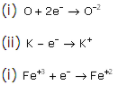(a) A solution of silver nitrate is good electrolyte but is not used for electroplating an article with silver because, the deposition of silver will be very fast and hence not smooth and uniform.
(b) (i) Reduction
(ii) Oxidation
(iii) Reduction

46. Choose A, B, C or D to match the descriptions (i) to (v) below . Some alphabets may be repeated.
(A) Non-electrolyte, (B) Strong electrolyte, (C) Weak electrolyte, (D) Metallic conductor
(i) Molten ionic compound
(ii) Carbon tetrachloride
(iii) An aluminium wire
(iv) A solution containing solvent molecules, solute molecules and ions formed by the dissociation of solute molecules
(v) A sugar solution with sugar molecules and water molecules.

(i) Molten ionic compound - Strong electrolyte
(ii) Carbon tetrachloride- Non-electrolyte
(iii) An aluminium wire- Metallic conductor
(iv) A solution containing solvent molecules, solute molecules and ions formed by the dissociation of solute molecules- weak electrolyte
(v) A sugar solution with sugar molecules and water molecules- Non-electrolyte

47. The following is an extract from metals in the service of man, Alexander and street /Pelican 1976': Alumina (aluminium oxide) has a very high melting point over 2000oC, so that I cannot readily be liquefied. However, conversion of alumina to aluminium and oxygen, by electrolysis, an occur when it is dissolved in some other substance.
(a) Which solution is used to react with bauxite as first step in obtaining pure aluminium oxide?
(b) The aluminium oxide for the electrolytic extraction of aluminium is obtained by heating aluminium hydroxide. Write the equation for this reaction.
(c) Name the element which serves both as the anode and the cathode in the extraction of aluminium.
(d) Write the equation for the reaction that occurs at the cathode during the extraction of aluminium.
(e) Write the equation for the reaction that occurs at the cathode during extraction of aluminium by electrolysis.
(f) Give the equation for the reaction that occurs at the anode when aluminium is purified by electrolysis.

(a) Bauxite is reacted with sodium hydroxide to obtain pure aluminium oxide.
(b) 2Al(OH)3 → Al2O3 + 3H2O
(c) Carbon serves both as anode and cathode.
(d) Reaction at cathode :During the electrolysis of molten lead bromide, which of the following takes place?
(a) Bromine is released at the cathode
(b) Lead is deposited at the anode
(c) Bromine ions gain electrons
(d) Lead is deposited at the cathode

(d) Lead is deposited at the cathode

49. (a) Here is an electrode reaction:
Cu → Cu+ + 2e-
At which electrode (anode or cathode) would such a reaction take place? Is this an example of oxidation?
(b) A solution contains magnesium ions (Mg+2), iron (II) ions (Fe+2) and copper ions (Cu+2). On passing an electric current through this solution which ions will be the first to be discharged at the cathode? Write the equation for the cathode reaction.
(c) Why is carbon tetrachloride, which is a liquid, a non - electrolyte?

(a) The reaction takes place at anode. Yes, this is an example of oxidation.
(b) Cu+2  will e discharged first.(c) carbon tetrachloride is a non - electrolyte as it is a covalent compound and contains only molecules.

50. The following is a sketch of an electrolytic cell used in the extraction of aluminium :(a) What is the substance of which the electrode A and B are made ?
(b) At which electrode (A or B ) is aluminium formed ?
(c) What are the two aluminium compounds in the electrolyte C ?
(d) Why is it necessary for electrode B to be continuously replaced ?

(a) Electrode A is made of gas carbon and electrode B is made of Carbon rods.
(b) At electrode A.
(c) Two compounds in the electrolyte are Al2O3 and Na3AlF6
(d) As at electrode B the oxygen is liberated during the process. The oxygen liberated oxidizes the carbon anode producing CO and CO2.Thus electrode B is to be replaced continuously.

51. A metal article is to be electroplated with silver. The electrolyte selected is sodium argentocyanide.
(a) What kind of salt is sodium argento cyanide?
(b) Why is it preferred to silver nitrate as an electrolyte?
(c) State one condition to ensure that the deposit is smooth, firm and long lasting.
(d) Write the reaction taking place at the cathode.
(e) Write the reaction taking place at the anode.

(a) It is a complex salt.
(b) Sodium argento cyanide is preferred over silver nitrate because it gives a smooth and firm silver plating over article.
(c) If silver nitrate is used then deposition of silver will be fast and not smooth.
(d) Reaction At Cathode :52. Find the odd one out from the following and explain your choice:
Al(OH)3, Pb(OH)2, Mg(OH)2, Zn(OH)2

Mg(OH)2 as it is basic while rest are amphoteric.

53. Correct the following statement:

54. Aqueous solution of nickel sulphate contains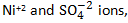(a) Which ion moves towards the cathode ?
(b) What is the product at the anode ?

(a) Nickel ions move towards cathode.
(b) Nickel ions.

55. Select the correct answer from the choices a,b,c and d which are given. Write only the letter corresponding to the correct answer.
(i) A compound which liberates reddish brown gas around the anode during the electrolysis in its molten state is :
(a) Sodium chloride
(b) Copper (II) oxide
(c) Copper (II) sulphate

(ii) During ionization metals lose electrons, this change can be called
(a) Oxidation
(b) Reduction
(c) Redox
(d) Displacement

(ii) Oxidation

56. Mr. Ramu wants electrolyte his key chain with nickel to prevent rusting. For this electroplating
(i) Name the electrolyte
(ii) Name the cathode
(iii) Name the anode
(iv) Give the reaction at the cathode
(v) Give the reaction at the anode

(i) Aqueous solution of nickel sulphate with few drops of dil. sulphuric acid
(ii) Article (e.g. key chain)
(iii) Pure nickel
(iv) Ni2+ + 2e-→ Ni
(v) Ni → Ni2+ + 2e-

57. Three different electrolytic cells, A,B, and C are connected in separate circuits. Electrolytic cell A contains sodium chloride solution. When the circuit is completed a bulb in the circuit glows brightly. Electrolytic cell B contains acetic acid solution and in this case the bulb in the circuit glows dimly. The electrolytic cell C contains sugar solution and the bulb does not glow. Give a reason for each of these observations

Cell A contains sodium chloride solution which is a strong electrolyte and contains only ions. So, it conducts electricity and the bulb glows brightly. Cell B contains both ions and molecules. So, there are few ions to conduct electricity and the bulb glows dimly.
Cell C contains sugar solution which is a non-electrolyte and does not contain ions. So, it is a bad conductor of electricity and the bulb does not glow.

58. Give reasons as to why - the electrolysis of acidulated water is considered to be an example of catalysis.

Dilute sulphuric acid catalyses dissociation, so electrolysis of acidified water is considered an example of catalysis.

59. Fill in the blanks from the choices given below :
(i) In covalent compounds, the bond is formed due to the ______ (sharing/transfer) of electrons.
(ii) Electro covalent compounds have a _____ (low/high) boiling point
(iii) A molecule of _____ contains a triple bond (hydrogen, ammonia, nitrogen).

(i) In covalent compounds, the bond is formed due to the sharing of electrons.
(ii) Electro covalent compounds have a high boiling point.
(iii) A molecule of nitrogen contains a triple bond.

60. Differentiate between electrical conductivity of copper sulphate solution and copper metal

 Copper sulphate solution Copper metal Conduction of electricity is due to the flow of ions. Conduction of electricity is due to the flow of electrons. It is an aqueous solution of an ionic compound. It is a metal in the solid state. It undergoes a chemical change. It remains unchanged chemically.

61. During the electrolysis of copper (II) sulphate solution using platinum as cathode and carbon an anode:
(i) What do you observe at the cathode and at the anode?
(ii) What change is noticed in the electrolyte?
(iii) Write the reactions at the cathode and at the anode.

(i) Red shiny metal is deposited at the cathode.
(ii) The colour of the electrolytes changes gradually from blue to colourless.
(iii) At the cathode:
Cu2+ + 2e→ Cu
Reaction at the anode:
OH→ OH + e-
4OH → 2H2O + O2

62. Copper sulphate solution is electrolyzed using copper electrodes Study the diagram given alongside and answer the questions that follows.
(i) Which electrode to your left or right is known as the oxidizing electrode and why?
(ii) Write the equation representing the reaction that occurs
(iii) State two appropriate observations for the above electrolysis reactions.

(i) The right electrode is the anode and oxidising electrode Cu → Cu2+ + 2elosing electrode.
(ii) Reaction at the anode: Cu → Cu2+ + 2e-
Reaction at the cathode: Cu2+ + 2e→ Cu
(iii) The anode dissolves and anode mud containing precious metal is recovered.

63. Which of these will act as a non-electrolyte?
(i) Liquid carbon tetrachloride
(ii) Acetic acid
(iii) Sodium hydroxide aqueous solution acid
(iv) Potassium chloride aqueous solution

(i) Liquid carbon tetrachloride

64. State one observation when electricity is passed through molten lead bromide.

Dark reddish brown fumes of bromine evolve at the anode and greyish white metal lead is formed on the cathode.

65. When fused lead bromide is electrolyzed we observe
(i) A silver grey deposit at anode and a reddish brown deposit at cathode
(ii) A silver grey deposit at cathode and reddish brown deposit at anode
(iii) A silver grey deposit at cathode and reddish brown fumes at anode
(iv) Silver grey fumes at anode and reddish brown fumes at cathode

(iii) A silver grey deposit at the cathode and reddish brown fumes at the anode.

66. The electrolyte used for electroplating an article with silver is:
(i) Silver nitrate solution
(ii) Silver cynide solution
(iii) Sodium argentocyanide solution
(iv) Nickel sulphate solution

(iii) Sodium argentocyanide solution

67. M is a metal above hydrogen in the activity series and its oxide has the formula M2O. The oxide when dissolved in water forms the corresponding hydroxide which is a good conductor of electricity. In the above context answer the following:
(i) What kind of combination exists between M and O?
(ii) How many electrons and there in the outermost shell of M?
(iii) Name the group to which M belongs
(iv) State the reaction taking place in the cathode
(v) Name the product at the anode

(i) Electrovalent or ionic compounds
(ii) One electron
(iii) Since it has valency 1, M belongs to Group 1.
(iv) At the cathode: M+ 1e→ M
(v) At the anode: Oxygen gas

68. Give appropriate scientific reasons for the following statements:
(i) Zinc oxide can be reduced to zinc by using carbon monoxide, but aluminium oxide cannot be reduced by a reducing agent
(ii) Carbon tetrachloride does not conduct electricity.
(iii) During electrolysis of molten lead bromide graphite anode is preferred to other electrodes
(iv) The electrical conductivity of acetic acid is less in comparision to the electrical conductivity of dilute sulphuric acid at a given concentration
(v) Electrolysis of molten lead bromide is considered to be a redox reaction

(i) Zinc is lower in the reactivity series, so it is comparatively less reactive. Hence, it is reduced by using carbon monoxide. But aluminium is very reactive; hence, it cannot be reduced by using a reducing agent and it can be reduced only by electrolytic reduction.
(ii) Carbon tetrachloride is a liquid and does not conduct electricity because it is a covalent compound and there are no free ions present and it contains only molecules.
(iii) In electrolysis of molten lead bromide, reactive bromine is liberated at the anode. As bromine is very reactive, an inert electrode like graphite is preferred in the electrolysis of molten lead bromide.
(iv) Acetic acid is a weak acid and has fewer ions, so conductivity is less, whereas dilute sulphuric acid is a strong acid and has more ions, and therefore, its electrical conductivity is more.
(v) During electrolysis of lead bromide, there is loss of electrons at the anode by bromine and gain of electrons at the cathode by lead. Thus, oxidation and reduction occur side by side. So, it is a redox reaction.
PbBr⇌ Pb+2 + 2Br-

69. Differentiate between the terms strong electrolyte and weak electrolyte (stating any two differences)

 Strong Electrolytes Weak Electrolytes Electrolytes which allow a large amount of electricity to flow through them. Electrolytes which allow small amounts of electricity to flow through them. These are good conductors of electricity. These are poor conductors of electricity. These almost completely dissociate in the fused or aqueous solution state. These are partially dissociated in the fused or aqueous solution state. These solutions contain only free mobile ions. These solutions contain ions as well as molecules.

70. (i) Copy and complete the following table :

 Anode Electrolyte Purification of copper

(ii) Write the equation taking place at the anode.

(i)

 Anode Electrolyte Purification of copper Impure copper Solution of copper sulphate and dilute sulphuric acid

(ii) Ag - e → Ag+
Cu - e- → Cu2+
Cl⁻ ‒ e→ Cl
Cl + Cl → Cl2

71. Give reasons why:
(i) Sodium chloride will conduct electricity only in fused or aqueous solution state
(ii) In the electroplating of an article with silver, the electrolyte sodium argento-cynide solution is preferred over silver nitrate solution
(iii) Although copper is a good conductor of electricity, it is a non-electrolyte.

(i) Electrostatic forces of attraction between ions in the solid state are very strong. These forces weaken in the fused state or in the solution state. Hence, ions become mobile.
(ii) If silver nitrate solution is used directly instead of double cyanide of silver and sodium, the deposition of silver will be very fast and hence not very smooth and uniform.
(iii) Copper has no mobile electrons in the solid state and an electrolyte should dissociate into oppositely charged ions to conduct electricity.
Hence, copper is a non-electrolyte.

72. (i) Name the product formed at the anode during the electrolysis of acidified water using platinum electrodes
(ii) Name the metallic ions that should be present in the electrolyte when an article made copper is to be electroplated with silver

(i) Oxygen is the product formed at the anode.
(ii) Ag+ and Na+

73. Write equations for the reactions taking place at the two electrodes (mentioning clearly the name of the electrodes) during the electrolysis of
(i) Acidified copper sulphate solution with copper electrodes
(ii) Molten lead bromide with inert electrodes

(i) Electrodes:
Cathode: Copper
Anode: Platinum
Reaction at the cathode: Cu2+ + 2e-→ Cu
Reaction at the anode: 4OH- - 4e-→ 4OH
2OH + 2OH →2H2O + O2

(ii) The cathode and anode are both made of graphite plates.
Reaction at the cathode: Pb2+ + 2e-→Pb
Reaction at the anode: Br- - e-→Br
Br + Br →Br2

74. Identify the substance underlined in each of the following cases:
(a) The electrolyte used for electroplating an article with silver.
(b) The particles present in a liquid such as kerosene, that is non-electrolyte.

(i) The electrolyte used for electroplating an article with silver: Sodium argentocyanide or potassium argentocyanide
(ii) The particles present in a liquid such as kerosene that is a non-electrolyte: Molecules

75. State the observations at the anode and at the cathode during the electrolysis of :
(a) Fused lead bromide using graphite electrodes
(b) Copper sulphate solution using copper electrodes

(i) Observations:
Anode: Dark reddish brown fumes of bromine evolve at the anode.
Cathode: Greyish white metal lead is formed on the cathode.

(ii) Observations:
Anode: Nothing gets deposited on the anode because the copper anode dissolves during the reaction as Cu2+ ions are formed.
Cathode: Reddish brown Cu is deposited.

76. Select the ion in each case, that would get selectively discharge from the aqueous mixture of the ions listed below :